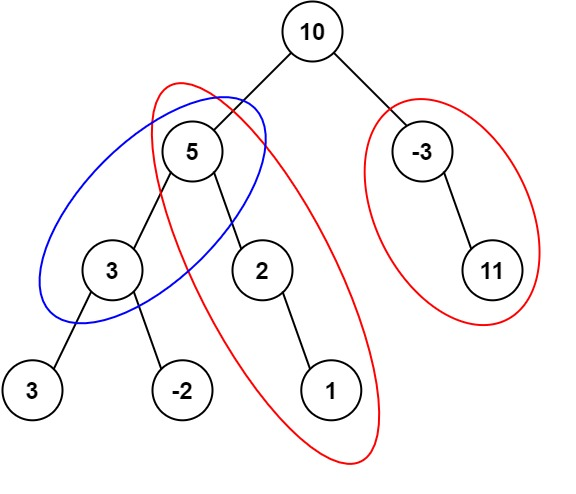### LeetCode• ㊗️
• 大家
• offer
• 多多！

## Problem

Given the root of a binary tree and an integer targetSum, return the number of paths where the sum of the values along the path equals targetSum.

The path does not need to start or end at the root or a leaf, but it must go downwards (i.e., traveling only from parent nodes to child nodes).

Example 1:Input: root = [10,5,-3,3,2,null,11,3,-2,null,1], targetSum = 8
Output: 3
Explanation: The paths that sum to 8 are shown.


Example 2:

Input: root = [5,4,8,11,null,13,4,7,2,null,null,5,1], targetSum = 22
Output: 3


## Code

/**
* Definition for a binary tree node.
* public class TreeNode {
*     int val;
*     TreeNode left;
*     TreeNode right;
*     TreeNode() {}
*     TreeNode(int val) { this.val = val; }
*     TreeNode(int val, TreeNode left, TreeNode right) {
*         this.val = val;
*         this.left = left;
*         this.right = right;
*     }
* }
*/
class Solution {
int res = 0;
int targetSum;
HashMap<Long, Integer> map;

public int pathSum(TreeNode root, int targetSum) {
map = new HashMap();
this.targetSum = targetSum;
help(root, 0);

return res;
}

public void help(TreeNode node, long currSum) {
if (node == null) return;

currSum += node.val;

if (currSum == targetSum) res++;

res += map.getOrDefault(currSum - targetSum, 0);

map.put(currSum, map.getOrDefault(currSum, 0) + 1);

help(node.left, currSum);
help(node.right, currSum);

map.put(currSum, map.get(currSum) - 1);
}
}

/**
* Definition for a binary tree node.
* public class TreeNode {
*     int val;
*     TreeNode left;
*     TreeNode right;
*     TreeNode() {}
*     TreeNode(int val) { this.val = val; }
*     TreeNode(int val, TreeNode left, TreeNode right) {
*         this.val = val;
*         this.left = left;
*         this.right = right;
*     }
* }
*/

class Solution {
public int pathSum(TreeNode root, int targetSum) {
if (root == null) return 0;

return (int)help(root, (long)targetSum) + pathSum(root.left, targetSum) + pathSum(root.right, targetSum);
}

private long help(TreeNode node, long targetSum) {
if (node == null) return 0;

return (node.val == targetSum ? 1 : 0)
+ help(node.left, targetSum - node.val)
+ help(node.right, targetSum - node.val);
}
}